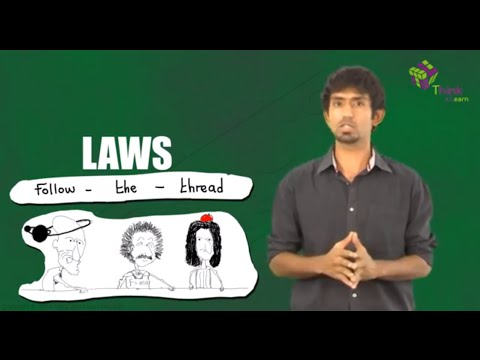# A massive disc of radius R is moved with a constant velocity m on a frictionless table. Another small disc collides with it elastically with a speed ofv0=0.3m/s, the velocities of the discs being parallel. The distance (shown in the figure) is equal to R/2, friction between the discs is negligible. For which u (in m/s) will the small disc move perpendicularly to its original motion after the collision?

### Elastic collission

An elastic collision is a collision in which there is no net loss in kinetic energy in the system as a result of the collision. Both momentum and kinetic energy are conserved in an elastic collision. In this case of collision, the kinetic energy before the collision and after the collision remains the same and is not converted to any other form of energy. In an elastic collision, the kinetic energy before the collision and after the collision remains the same. It is not converted to other forms of energy.

V0y=V0sinθ
This component does not change throughout
Also from the given data
v0x=v0cosθ
ucosθ
For elastic collision relative velocity before collision is equal to relative velocity after collision along x−axis

v0cosθ−ucosθ=ucosθ+vx

If

vxcosθ=v0ySinθ

Hence

m=0.1

Check out the video given below for more informationArticles to explore(0)(0)